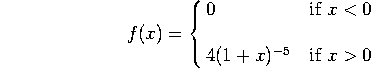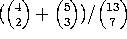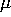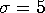Practice M362K Final

Originally given in December 1991

Note: This is only 6 of the 7 problems given in that exam. The remaining problem depended on a handwritten table that obviously didn't get stored on disk. This is the exam from F91 when the class was based on a different (terrible!) textbook. I did not do a very good job teaching that class (it was my first term here), and we didn't get as far as we should have. As a result, the final was somewhat more elementary than ours is likely to be.

Problem 1. Baseball

Cal Ripken plays shortstop for the Baltimore Orioles (my favorite baseball team). Suppose that every time he comes up to bat he has a 30% chance of getting a hit (independent of how he does in all his other times at bat). Suppose he comes up to bat 500 times in 1992.

a) What is the probability that he will finish the season with a batting average of .330 or better (i.e. that he will get 165 or more hits)? [For this part of the problem I want an EXACT answer (that is, some sort of formula), not a numerical approximation.]

b) Use the normal approximation to numerically approximate this probability.

c) Using the normal approximation, estimate the probability that he finishes the season with a batting average of EXACTLY .330 (remember the continuity correction!).

Problem 2. Continuous distributions

The lifetime of a certain brand of light bulb is a random variable (call it X), with probability density functiona) Find the mean and the standard deviation of X.

b) What is the probability that a randomly chosen lightbulb lasts less than a year?

c) Suppose we buy 5 lightbulbs. What is the probability that exactly 3 of these bulbs will burn out in the next year?

Problem 3. Conditional Probability

A system consists of 4 components (call them A, B, C, and D). On a given day, each component has a 50% probability of failing (independent of the others). If 3 or more components fail, the system breaks down.

a) What is the probability of the system breaking down today?

b) Given that it broke down yesterday, what is the probability that component A failed?

Problem 4. Bridge

A bridge hand consists of 13 cards. For this problem you do not have to numerically evaluate your results. An answer likeis just fine.

a) What is the probability that a bridge hand (13 cards) contains exactly 5 spades?

b) What is the probability that a bridge hand contains exactly 5 spades AND exactly 5 hearts?

c) What is the probability that a bridge hand contains exactly 5 spades OR exactly 5 hearts (or both, of course)?

Problem 5. French Fish

Alain, Blaise, and Camille go fishing at Lac du Traute, somewhere in France. In France, catching fish is a Poisson process, with mean 0.15 fish/hour (per person). They spend 8 hours fishing on the lake.

a) What is the probability that Alain catches at least one fish in the first 4 hours?

b) What is the probability that Blaise catches exactly 2 fish in the day?

c) What is the probability that the 3 friends (they ARE friends) go home with a total of 3 fish?

Problem 6. Going Bananas

It's impossible to fill a banana crate to exactly a specified weight. If you aim for weight, you wind up with a normal distribution of weights with meanand standard deviationpounds.

a) What weight should the crate-fillers aim for in order to insure that 99% of the crates weigh at least 100 pounds?

b) Suppose the mean is set to be 110 pounds. A zookeeper buys 4 crates. What is the probability that he will get 430 pounds or more?